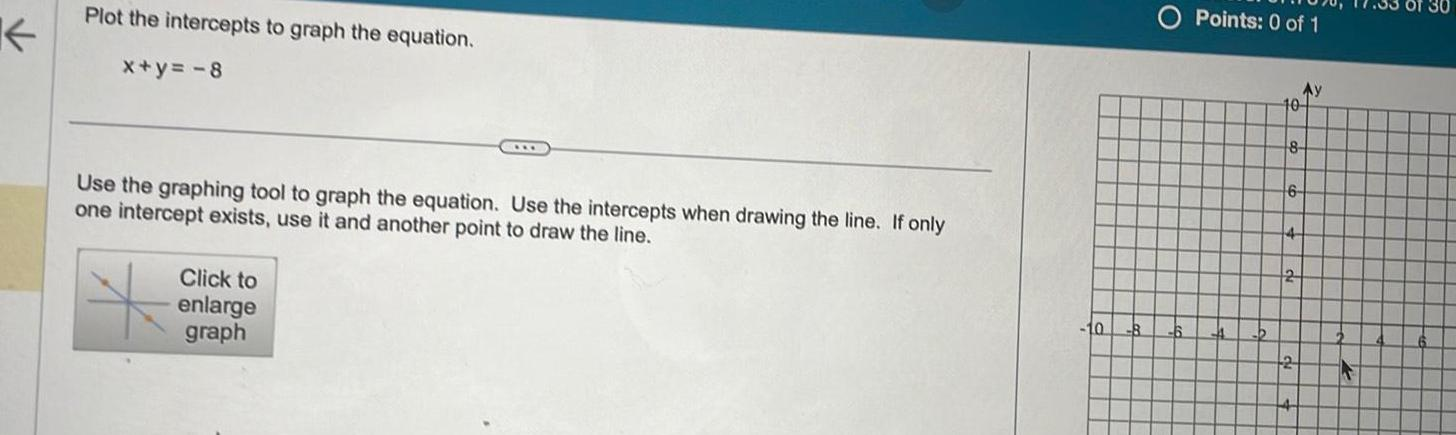Question:

# Plot the intercepts to graph the equation x y 8 Use the

Last updated: 9/18/2023Plot the intercepts to graph the equation x y 8 Use the graphing tool to graph the equation Use the intercepts when drawing the line If only one intercept exists use it and another point to draw the line Click to enlarge graph O Points 0 of 1 Ay 10 8 6 2